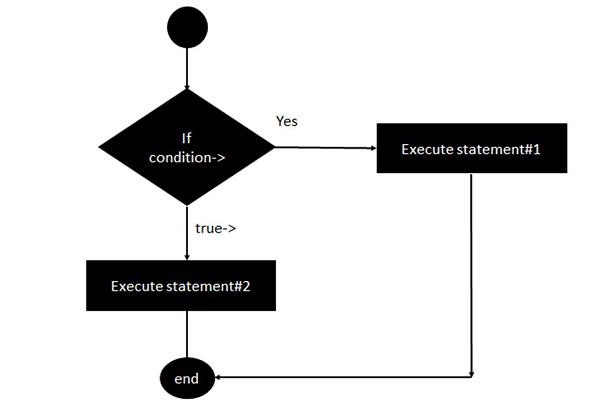# Erlang - If statement

The first decision making statement we will look at is the ‘if’ statement. The general form of this statement in Erlang is shown in the following program −

## Syntax

```if
condition ->
statement#1;
true ->
statement #2
end.
```

In Erlang, the condition is an expression which evaluates to either true or false. If the condition is true, then statement#1 will be executed else statement#2 will be executed.The following program is an example of the simple if expression in Erlang −

## Example

```-module(helloworld).
-export([start/0]).

start() ->
A = 5,
B = 6,

if
A == B ->
io:fwrite("True");
true ->
io:fwrite("False")
end.
```

The following important things need to be noted about the above program −

• The expression being used here is the comparison between the variables A and B.

• The -> operator needs to follow the expression.

• The ; needs to follow statement#1.

• The -> operator needs to follow the true expression.

• The statement ‘end’ needs to be there to signify the end of the ‘if’ block.

The output of the above program will be −

## Output

```False
```
erlang_decision_making.htm